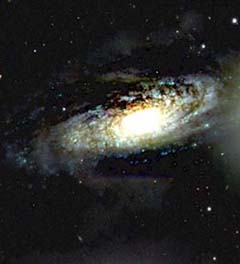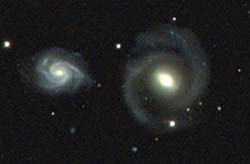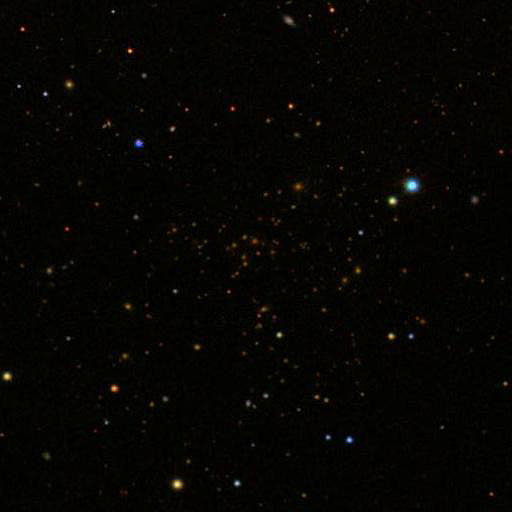In Section I, you used SkyServer to look up redshifts of twelve galaxies. In this section, you will learn how to calculate redshifts for yourself.

If any of the following words or concepts are not familiar to you, read about them in any introductory physics or astronomy text before you begin this section:

• spectrum, spectra
• lines (absorption, emission)
• wavelength
• Angstrom
• Balmer series
• Doppler shift

Astronomers learn an amazing number of things from the analyzing the spectra of stars, galaxies, and quasars. In this section, we will focus on just one application: we will learn how to measure the redshift of a galaxy from its spectrum, and we will learn how to interpret and use this number.

## Measuring Redshifts

Measuring a redshift or blueshift requires four steps:

1. obtain the spectrum of something (let’s say a galaxy) that shows spectral lines
2. from the pattern of lines, identify which line corresponds to which atom, ion, or molecule
3. measure the shift of any one of those lines with respect to its expected wavelength, as measured in a laboratory on Earth
4. apply a formula that relates the observed shift to velocity along the line-of-sight

An example will help to show how this works. Hydrogen is the most abundant element in the universe, and it is often seen in galaxies where gas becomes ionized and fluoresces. The spectrum of such a region shows a pattern called the Balmer series of lines in emission. The Balmer lines for emission are easy to reproduce in a classroom with a hydrogen discharge tube. The energizing agent that makes the gas glow is not the same as in galaxies, but the spectrum – the pattern of lines – is the same. Either from your own measurements in the classroom, or from pre-tabulated information, the rest wavelengths of the Balmer lines are as follows:

Rest Wavelengths of Hydrogen – Balmer Series
Name Color Wavelength (Angstroms)
Alpha (a) Red 6562.8
Beta (b) Blue-green 4861.3
Gamma (g) Violet 4340.5
Delta (d) Deep Violet 4101.7
Exercise 14: Using SkyServer, look at the spectrum of the object with ID = 1237666407379828976. You can find the spectrum using the Get Spectra Tool, in Plate 401/51788, Fiber 161, and it is reprinted below.

This spectrum comes from a galaxy, and like many others, it shows strong emission lines. The hydrogen lines are already identified for you: the tallest peak is the a line, and the tall peak to its left is the b line. Once the pattern becomes familiar to you, you should be able to recognize these lines even in unmarked spectra.

Read off the wavelengths of the Balmer lines on the x-axis of the spectrum to verify the entries in this table:

Wavelengths of Hydrogen – Balmer Series for
Object ID # 1237666407379828976
Name Color Wavelength (Angstroms)
Alpha (a) Red 7220
Beta (b) Blue-green 5360
Gamma (g) Violet 4780
Delta (d) Deep Violet 4500

The redshift, symbolized by z, is defined as:

`1 + z = lobserved / lrest.`

For example, taking the Balmer gamma line,

`1 + z = 4780 / 4340.5 = 1.1, so`
`z = 0.1.`

Note that if the observed wavelength were less than the rest wavelength, the value of z would be negative – that would tell us that we have a blueshift, and the galaxy is approaching us. It turns out that almost every galaxy in the sky has a redshift in its spectrum.

Choosing the alpha, beta, or delta lines would also yield z = 0.1 – the measured redshift does not depend on which line you choose. If this statement is found not to be true (within the errors of measurement, of course), then most likely you have not made the correct identification of at least one of the lines.

## Interpreting Redshifts

You have just directly computed the redshift for a galaxy. The quantity z is dimensionless, and since it is derived directly from data, its value is unambiguous. We will often use just this number. However, sometimes we want to express the result as the velocity of the galaxy with respect to us, in units of km/sec.Converting from redshift z to velocity v measured in km/sec is easy – the formula is

`v = c z ,`

where c is the speed of light, c = 3 x 105 km/sec.

Thus, in this example, galaxy 1237666407379828976 appears to be moving away from us at 0.1 x 3 x 105 km/sec = 30,000 km/sec. This value is typical of the galaxies found in the SkyServer database.

Since the formula is equivalent to z = v / c, it contains an interpretation of the meaning of the value of z: z measures the galaxy’s speed of recession relative to the speed of light.

Up to this point things are straightforward, but there are two important qualifications. First, the formula v = c z is accurate only when z is small compared to 1.0 (0.1 would be OK in this sense). For high velocities, those that approach the speed of light, a more complicated formula is needed to derive an accurate velocity v from the measured redshift z. Second, while we often speak of the “recession of galaxies,” which implies motion through space, in fact the expanding universe picture is that space itself is expanding: the galaxies are not moving through space, but just being carried along by space as it expands (see The Hubble Diagram for more about this concept). In this picture, the redshift of a galaxy is not supposed to be interpreted as a velocity at all, even though the observed redshift looks just like a Doppler shift.

Rather, in this cosmological context, the redshift tells us the relative scale of the universe at the time the light left the galaxy. Suppose the distance to galaxy 1237666407379828976 was d(z) at the time the light that we are now observing left it (to give some perspective, for z = 0.1, this time was roughly a billion years ago). In those billion years, the space in the universe has expanded, so that now the separation between our galaxy and galaxy 1237666407379828976 is d(0). Then

`1 + z = d(0) / d(z) .`

We interpret this formula as follows: at the time corresponding to redshift z = 0.1, all galaxies were 10% closer together. We can also say that the universe has stretched by the same factor as have the wavelengths. A measured value of z = 0.2 corresponds to a time when galaxies were 20% closer together than they are now, and so on.

Question 6: In the SkyServer database, you can find redshifts for quasars such that z > 1. Is there a conceptual problem if the redshift is interpreted as a Doppler shift velocity? Is there a conceptual problem if the redshift is interpreted as the cosmological stretching of space?

Read this section only if you want to dig deeper into the interpretation of z. You can skip directly to Exercise 15 if you wish. There are really two kinds of redshifts, each with its own interpretation. Some redshifts are dynamic – they arise from moving objects (for example, two stars in orbit around each other); other redshifts arise from the cosmological expansion of space described above. If you are observing stars, the Doppler interpretation of redshift is completely adequate. You will also rarely need to worry about the accuracy of the formula v = c z because v is almost always small compared to c.Galaxies also have dynamic motions with respect to their neighbors – binary galaxies orbit one another, and galaxies have more complicated orbits within groups and clusters. Single galaxies can feel the gravitational tug of neighboring masses, and can move through space as a result of the gravity. All these velocities are also much smaller than the speed of light, and you can use v = c z. Once again, in cases of galactic motion, the Doppler interpretation is OK.

In the cosmological application, we assume that the random motions of galaxies cancel to zero in some volume. When we say something like: “the redshift of the galaxy reflects the expansion of space,” we are assuming that the galaxy is at rest with respect to this volume; that is, the redshift arises only from the cosmological expansion of space.

In reality, though, the redshift of any galaxy will have two components: a dynamic component and a cosmological component. However, from Earth we can measure only a single number, the redshift z. Without external arguments, we cannot distinguish the two types of redshifts. As a general rule, for nearby galaxies (z < 0.001), the cosmological component is small: the dynamic part prevails and we can think in terms of Doppler shifts (objects moving through space). For relatively distant galaxies (z > 0.01), the dynamic part is smaller than the cosmological part, and thinking in terms of Doppler shift velocities could be misleading. At intermediate redshifts, z ~ 0.003, the two contributions to the measured redshift can be comparable in size. In this case, sorting out what is what is a challenge even to experts.

Exercise 15: Not all galaxies have spectra with strong emission lines. Moreover, even though hydrogen is by far the most common element in the universe, it does not necessarily follow that hydrogen’s spectral lines (the Balmer lines) will be the strongest lines seen in a spectrum of a galaxy. To help recognize more complicated patterns of lines seen in galaxies, it helps to build a set of example spectra that seem to be characteristic of a class. Examples of galaxy classes include those with strong emission lines, those with no emission lines but strong absorption features, and those with moderate amounts of both. Astronomers can match these examples, called template spectra, to the spectra of galaxies at unknown redshifts, and move the spectra to find the redshifts. The SDSS uses nine template spectra.

The Redshifts app lets you use SDSS’s templates to find the redshifts of ten galaxies. Select the spectrum you wish to look at with the drop-down menu labeled “spectrum.” Select the template you wish to compare it to with the drop-down menu labeled “template.” Compare each spectrum to the nine templates to find the one it most resembles. Then, use the left, right, step forward (>>) and step backward (<<) buttons at the bottom of the page to move the unknown spectra left and right. Above the spectrum, the application shows the redshift you are testing.

When the peaks and valleys of the unknown spectrum match with the peaks and valleys of one of the templates, you have found the redshift of the unknown spectrum. Don’t try to match the entire spectrum perfectly; just get the major peaks and valleys to match. When you find the redshift that matches the spectrum most closely, write the spectrum number from the drop-down menu, then write the redshift you found.

## Redshifts of Sample Galaxies

Now that you know what redshift is and how to measure it, you are ready to return to the sample galaxies in the three clusters from the last section.Exercise 16: Open your online notebook containing the galaxies you found relative distances to in the last section. Of the objects you selected, at least four should have spectra available in the SDSS spectra database. In fact, these four were among the ten galaxies you found redshifts for in the last exercise.

The table below tells you which spectrum number from Exercise 15 corresponds to which galaxy’s object ID from the Distances section. Click any of the object IDs to open the Object Explorer in the tools window. Write down the redshift (the “z” just above the spectrum) for each galaxy.

Spectrum Number Galaxy ID
527 1237671126979052049
530 1237671126979051781
523 1237671126979051779
525 1237671126979051984

Compare the redshifts found by the SDSS with the redshifts you calculated in Exercise 13. How close were you?

Exercise 17: What is the average redshift of the galaxies in Exercise 16? What is the average redshift in the SkyServer database? To find out, pick a few dozen galaxies at random from the database. Find the average redshift, and plot a histogram of redshifts. What is the characteristic range of redshifts? Do the same for objects classified as quasars – what is their mean redshift, and what is the range?

The easiest way to examine several spectra at once is to use the Plate Browser tool. Choose any plate from the dropdown menu, then click on any of the “galaxy” links to see a spectrum. The redshift is the “z” value written at the bottom of each spectrum.